Click to Chat

1800-1023-196

+91-120-4616500

CART 0

• 0

MY CART (5)

Use Coupon: CART20 and get 20% off on all online Study Material

ITEM
DETAILS
MRP
DISCOUNT
FINAL PRICE
Total Price: Rs.

There are no items in this cart.
Continue Shopping```Minors and Co-FactorsThe minor of an element of a determinant is again a determinant (of lesser order) formed by excluding the row and column of the element. For example take the following determinant

Δ =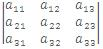If we leave the row and column passing through aij (aij means the element belonging ith row and jth column) then we obtain a second order determinant which is minor of aij and is denoted by Mij. In general, minor Mij of an element aij is the determinant excluding ith row and jth column. Thus we have 9 minors corresponding to 9 elements of above determinant Δ. Here we are illustrating some minors of the determinant Δ.

(i) The minor of element a11 = M11 =(ii) The minor of element a22 = M22 =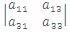(iii) The minor of element a31 = M31 =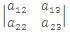and so on.

Cofactor of an element aij is defined as Cij = (-1)i+j Mij.

Where, Cij = cofactor of aij.

Illustration:

Find the minors and cofactors ofalong second column.

Solution:

Minors along second column i.e. elements 2, 5 and 8 areAnd cofactors of the corresponding elements are

C12 = (-1)1+2 (-6) = 6

C22 = (-1)2+2 (-12) = -12

C32 = (-1)3+2 (-6) = 6 respectively.

Evaluation of a Determinant

The determinant of order m can be evaluated as

Δ  = ∑mi=1  aij .Cij, j = 1, 2, ...... m.

= ∑mj=1  aij.Cij, i = 1, 2, ...... m.

i.e. the determinant can be evaluated by multiplying the elements of a single row or a column with their respective co-factors and then adding them.

For e.g.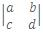Cofactor of a = d(-1)1+1 = d

Cofactor of b = c(-1)2+1 = -c

So, the value of the determinant is (ad - bc).

Illustration:

Expand the following determinant.

Δ =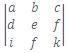= ∑mi=1  aij cij

Solution:

.·. Δ = a.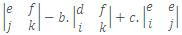a(ek - f2) -b (dk - if) +c (dj - ie)

= a M11 - b M12 + c M13 = a C11 + b C12 + c C13

Note : Though in the example, elements of first row and their cofactors are considered, the value of the determinant can be evaluated from any row and column.

Sarrus Rule: Sarrus give a rule for a determinant of order 3. Write down the three rows of a determinant and rewrite the first two rows. The three diagonals sloping down the right give the three positive terms and the three diagonals sloping down to the left give the three negative terms.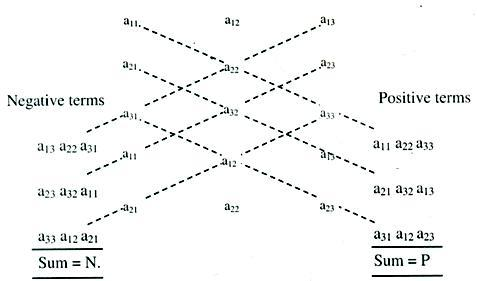.·. Δ = P - N.

IIT JEE study material is available online free of cost at askIITians.com. Study Physics, Chemistry and Mathematics at askIITians website and be a winner. We offer numerous live online courses as well for live online IIT JEE preparation - you do not need to travel anywhere any longer - just sit at your home and study for IIT JEE live online with askIITians.com

To read more, Buy study materials of Matrices and Determinants comprising study notes, revision notes, video lectures, previous year solved questions etc. Also browse for more study materials on Mathematics here.
```### Course Features

• 731 Video Lectures
• Revision Notes
• Previous Year Papers
• Mind Map
• Study Planner
• NCERT Solutions
• Discussion Forum
• Test paper with Video Solution